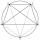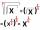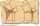Tangents

To circle with a radius of 41 cm from the point R guided two tangents. The distance of both points of contact is 16 cm. Calculate the distance from point R and circle centre.

Result

d =  41.8 cm

Solution:Leave us a comment of example and its solution (i.e. if it is still somewhat unclear...):Be the first to comment!Next similar examples:

1. Concentric circlesIn the circle with diameter 19 cm is constructed chord 9 cm long. Calculate the radius of a concentric circle that touches this chord.
2. ThalesCalculate the length of the Thales' circle described to right triangle with hypotenuse 18.4 cm.
3. 10 piecesHow to divide the circle into 10 parts (geometrically)?
4. Triangle ABCRight triangle ABC with right angle at the C, |BC|=18, |AB|=33. Calculate the height of the triangle hAB to the side AB.
5. Eq with reciprocalSolve given equation with reciprocal member: a-6/a+10=4/8
6. Theorem proveWe want to prove the sentence: If the natural number n is divisible by six, then n is divisible by three. From what assumption we started?
7. Completing squareSolve the quadratic equation: m2=4m+20 using completing the square method
8. EquationEquation ? has one root x1 = 8. Determine the coefficient b and the second root x2.
9. RootsDetermine the quadratic equation absolute coefficient q, that the equation has a real double root and the root x calculate: ?
10. Square rootsWhat is equal to the product of the square roots of 295936?Find the roots of the quadratic equation: 3x2-4x + (-4) = 0.Determine the discriminant of the equation: ?The product of two consecutive odd numbers is 8463. What are this numbers?How much is sum of square root of six and the square root of 225?Solve quadratic equation: 2x2-58x+396=0Solve quadratic equation: ?Calculate height and sides of the right triangle, if one leg is a = 81 cm and section of hypotenuse adjacent to the second leg cb = 39 cm.